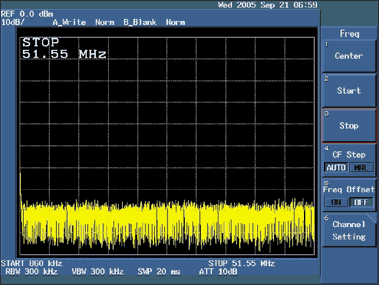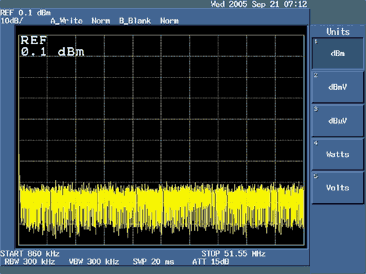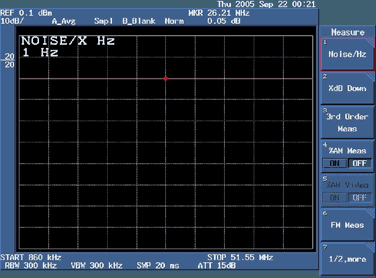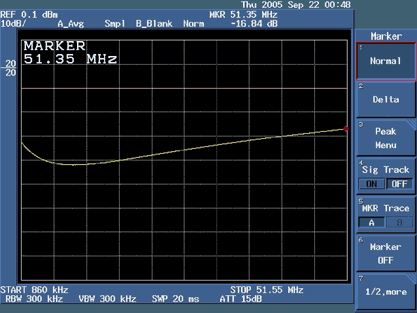# Measuring Return Loss Using the Advantest R3132 Spectrum Analyzer

### Abstract

This application note contains the basic operating procedures needed to help engineers measure return loss using the Advantest® R3132 spectrum analyzer (or similar spectrum analyzer), and should give them enough information to measure return loss in the laboratory. While the specific key-press operations apply only to the R3132, the basic procedures can be useful for all spectrum analyzers. It is not an all-encompassing tutorial, and some basic knowledge of spectrum-analyzer operation is needed.

### Return-Loss Requirements

For E3, ITU G.703, and ETS 300-686, specify the input return-loss requirements shown in Table 1 and the output return-loss requirements shown in Table 2.

Table 1. Input-Port Minimum Return Loss

 Frequency Range (kHz) Return Loss (dB) 860 to 1720 12 1720 to 34,368 18 34,368 to 51,550 14

Table 2. Output-Port Minimum Return Loss
 Frequency Range (kHz) Return Loss (dB) 860 to 1720 6 1720 to 51,550 8

### Measuring Return Loss on Dallas Semiconductor LIUs

The test setup and procedures for measuring E3 return loss are described in subclauses A.2.5 and A.2.6 of the ETS 300-686 specification. The test configuration in Figure 1 is designed to measure the input return loss and verify compliance with the requirements shown in Table 1. The output return-loss configuration is similar, with the same equipment connected to the transmitter output instead of the receiver input.Figure 1. Return-loss measurement setup.

In the Dallas Semiconductor setup, a Wide Band Engineering (WBE) Company, Inc. return-loss bridge (part number A57TLSTD) is used. To interface the 75Ω bridge with the 50Ω generator and 50Ω spectrum-analyzer ports, two WBE 50Ω/75Ω impedance converters (part number A65L) are used. The precision 75Ω resistor to the right of the bridge in Figure 1 is built into the return-loss bridge. The Advantest R3132 spectrum analyzer serves as both the signal generator and the spectrum analyzer in Figure 1.

In the setup shown in Figure 1, the generator is supplying a sinusoidal 1V-peak signal at frequencies ranging from 860kHz to 51,550kHz.

To check the test setup before making return-loss measurements, the NTP interface of the bridge (the interface on the left in Figure 1) should be connected to a 75Ω (± 0.25Ω) test load. In the Dallas Semiconductor setup, this precision resistor is a component from WBE that comes with the return-loss bridge. With this test load, the return loss should be 20dB higher than the requirements shown in Table 1.

### Spectrum Analyzer Main Functions

The main functions needed to make a return-loss measurement can be broken down into six categories:

1. Frequency setup
2. Reference level setup
3. Bandwidth, sweep, trigger control setup
4. Tracking generator setup
5. Trace control setup
6. Using marker to measure return loss in dB

### Frequency Setup

The following procedure is used to configure the frequency range of the spectrum analyzer:

1. Press the FREQ key on the front panel.
2. Press soft key #2 (Start) and type the start frequency using the numeric keypad, followed by the appropriate units (GHz, MHz, kHz, or Hz) key to enter the value. From Tables 1 and 2, for both the input and output port, the frequency range starts from 860kHz, as shown in Figure 2.Figure 2. Setting up the start frequency at 860kHz.

3. Press soft key #3 (Stop) and type the stop frequency using the numeric keypad, followed by the appropriate units (GHz, MHz, kHz, or Hz) key to enter the value. From Tables 1 and 2, for both the input and output port, the frequency range ends at 51.55MHz, as shown in Figure 3.Figure 3. Setting up the end frequency at 51.55MHz.

4. The remaining settings can be left in the default state.

### Reference Level Setup

The following procedure is used to configure the reference level of the spectrum analyzer:

1. Press the LEVEL key on the front panel.
2. Press soft key #2 (ATT) and select AUTO if it is not already selected.
3. Press soft key #3 (dB/div) to select dB/div display type. Select soft key #1 (10dB/div) as the desired decibels per division ratio, as shown in Figure 4 and then press the RETURN key.Figure 4. Selecting 10dB/div as the desired decibels-per-division ratio.

4. Press soft key #5 (Units) and select soft key #1 (dBm) as the desired display units, as shown in Figure 5 and then press the RETURN key.Figure 5. Selecting dBm as the desired display units.

5. Press soft key #1 (Ref Level) and type the desired level using the numeric keypad. You can also use the data knob to select the desired level. We used 0.1dBm to measure the return loss.
6. The remaining settings can be left in the default state.

### Bandwidth, Sweep, and Trigger Control Setup

The following procedure is used to configure the bandwidth, sweep, and trigger control of the spectrum analyzer:

1. Press the BW key on the front CONTROL panel.
2. Press soft key #7 (Auto All) to set the resolution and video bandwidth automatically.
3. Press the SWEEP key on the front CONTROL panel.
4. Press soft key #2 (Auto All) to set the sweep time automatically.
5. Press soft key #6 (Gated Sweep) and select OFF if it is not already selected.
6. Press soft key #7 (Ext Gate In) and select OFF if it is not already selected.
7. Press the TRIG key on the front CONTROL panel.
8. Press soft key #1 (Trig Source) and select Free Run if it is not already selected. Press the RETURN key.
9. The remaining settings can be left in the default state.

### Tracking Generator Setup

The following procedure is used to configure the tracking generator output of the spectrum analyzer:

1. Press the TG key on the front panel.
2. Press soft key #1 (TG Level) and type the desired output signal level using the numeric keypad, followed by the appropriate units (+dBm or -dBm) key to enter the value. The data knob can also be used to adjust the output signal level. To measure return loss, we used 0.0dBm.
3. Press soft key #4 (Ref Line) to set the reference line level that is used to normalize the input signal. Type in the desired reference line level using the numeric keypad, followed by the appropriate units (+dBm or -dBm) key to enter the value. The data knob can also be used to adjust the output signal level. To measure return loss, we used -20dBm.
4. Press soft key #3 (Norm Corr) and select OFF if it is not already selected. Disabling the normalization function allows a new set of correction data to be saved in the next step.
5. Press soft key #2 (Execute Normalize) to save correction data and normalize the input signal to the reference line level.
6. The remaining settings can be left in the default state.

### Trace Control Setup

The following procedure is used to configure the tracking generator output of the spectrum analyzer:

1. Press the TRACE key on the front CONTROL panel.
2. Press soft key #1 (Write A) to display the input signal trace.
3. Press soft key #5 (Detector) and select the desired signal trace detection method (Normal, Posi, Negi, or Sample). Normal displays both positive and negative peak values. Posi displays positive peak values. Negi displays negative peak values. Sample displays the current signal trace. To measure return loss, use soft key #4 (Sample) method as shown in Figure 6. Press the RETURN key.Figure 6. Selecting the Sample method to display the current signal trace.

4. Press soft key #7 (1/2, more) to display the next set of soft keys. Use soft key #1 (AVG A) to keep it in ON mode. Press the RETURN key. Press soft key #7 (2/2, more) again to return to the original set of soft keys.
5. The remaining settings can be left in the default state.

### Measuring Return Loss in dBm

1. Press the MEAS key on the front MARKER panel.
2. Press soft key #1 (Noise/Hz) as shown in Figure 7.Figure 7. Selecting Noise/Hz for return loss measurement setup.

3. Press soft key #1 (dBm/Hz).Figure 8. Selecting dBm/Hz for return-loss measurement setup.

4. Press soft key #7 (Noise/Hz OFF), as shown in Figure 8.
5. Place the 75Ω termination into the bridge and measure the dB.Figure 9. Return Loss with 75Ω termination.

6. Use the MKR key from the front MARKER panel. Then use the data knob to go to the frequency of interest to measure return loss at that frequency, as shown in Figure 9.
7. Now connect the part of the device under test (DUT) to the bridge and use the data knob to go to the frequency of interest. For return loss, we will measure dB at 860kHz, 1.720MHz, 34.3680MHz, and 51.55MHz. We will use the data knob to go to one of these frequencies. The user can also use the data knob to go to the frequency of interest to measure dB at that frequency.
8. Measure the return loss at 860kHz for the DUT, as shown in Figure 10.Figure 10. Return loss of the DUT at 860kHz.

9. Measure the return loss at 1.72MHz for the DUT, as shown in Figure 11.Figure 11. Return loss of the DUT at 1.72MHz.

10. Measure the return loss at 34.368MHz for the DUT, as shown in Figure 12.Figure 12. Return loss of the DUT at 34.37MHz.

11. Measure the return loss at 51.35MHz for the DUT, as shown in Figure 13.Figure 13. Return loss of the DUT at 51.35MHz.